# Set Identity function

The set Identity function and related functions are discussed below.

## What is Set Identity function?

The function that associates each real number to itself is called the identity function and is denoted by the II(x) = x : xR

## Domain and range of the Set Identity function

The domain and range of the identity function are both equal to R. the graph is a straight line passing through the origin and inclined at an angle ofwith an x-axis
F(x) = x
F(1) = 1
F(2) = 2

## Modulus Functions

If f(x) = |x| = {x when x ≥ 0,
-x when x < 0} Is called the modulus function or absolute value function the domain of the modulus function is the set of all real numbers & range is the set of all nonnegative real number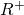= {xR : x ≥ 03} (1) If x ≥ 0 the graph coincide. With the graph of the identity functional (g= x) If x < 0 it coincides with the line y = -x

## Properties of Modulus Function

1) For any real number x= |x|

2) a,b is a positive real number, then|x| ≤ a -a ≤ x ≤ a|x| ≥ a x ≤ -a or x ≥ a<|x| < a -a < x < a>|x| > a x < -a or x > a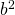a≤ |x| ≤ b x[-b, -a] u [a,b]<<a ≤ |x| ≤ b[-b, -a] u [a,b] 3) for real number x and y |x+y| = |x| + |y| (x≥0 and y≥ 0) or (x< 0 and y< 0) |x-y| = |x| – |y| (x≥0 and |x|≥ |y|) or (x≤ 0 , y≤ 0 and |x| ≥ |y|) |x± y| ≤ |x| + |y| |x± y| ≥ |x| – |y|

## Greatest Integer Function (Floor Function)

f(x) = [x] for all xεR or ⌊x⌋ is called greatest integer function

for any real number x the smallest [x] to denote the greatest integer less than or equal to x
The domain of the greatest integer function is the set of R to all real numbers and the range is the set of Z of all integers as it attains only integer value.

e.g. [2.75] = 2, = 3, [0.74] = 0, [-7.45] = -8 etc

Properties:- if n is an integer and x is a real number between n and n+1 then

[-n] = -[n]
[x + k] = [x] + k for any integer k
[-x] = -[x] -1

[x] + [-x] =[x] – [-x] =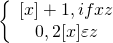[x] ≥ k = x ≥ k where kz
[x] ≤ k = x < k where kz [x] > k = x ≥ k +1 where kz
[x] < k = x < k where kz [x+y] = [x] + [y+x-[x] for all x,yR= [n x], nN

## Smallest Integer Function

f(x) = ⌈x⌉ for all x εR
Is called the smallest integer function or the ceiling function.

The domain of the smallest integer function is the set of R of all real numbers and its range is the set z 0 < all integers

e.g:- ⌈4.75⌉=5, ⌈-7.2⌉ = -7,⌈5⌉=5, ⌈.75⌉=1, etc.

Properties of Smallest Integer Function ⌈-n⌉= -⌈n⌉ ,nz ⌈-x⌉= -⌈x⌉+ 1,xR-z ⌈x+n⌉= ⌈x⌉+ n,xεR-z and nz ⌈x⌉+ ⌈-x⌉ =⌈x⌉- ⌈-x⌉ =## Fractional Part Function

The function f(x) = {x} for all xεR
The symbol {x} denotes the fractional part of or decimal part of x.
The domain of the fractional part function is the set of R of real numbers and the range is the set [0,1]
f(x) = {x} = x – [x] : x εR
e.g. 3.45 = 0.45, [2.75] = 0.25, [-0.55] = 0.45,  = 0, [-7] = 0 etc

## Signum Function

If f(x) =.3 × .3 =.09
f(x) =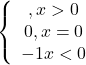The domain of the signum function is the set of R (all real numbers) and the domain is the set (-1,0,1)

## Exponential Function

F (x) =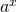where a> 0 and a ≠ 1
If a > 1
If y = f(x) =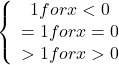We observe that –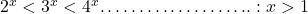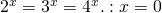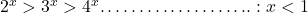If 0 < a<1 Y = f(x) =decrease with the increase in x. And y>0 : x ε R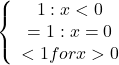if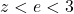then=”” graph=””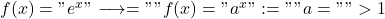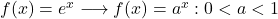</e<3\$>